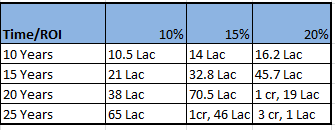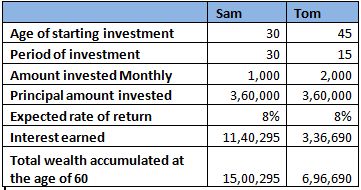## FinAtoZ BlogTo become a good investor, one first need to understand and appreciate how power of compounding can create exponential returns in the long run. A simple concept that all of us learnt in mid school, works wonders when it comes to growth in wealth.

Albert Einstein once said: “Compound interest is the eighth wonder of the world. He who understands it, earns it … he who doesn’t … pays it.”

First lets try to understand the concept of Compounding.

## The Snow Ball EffectCompounded Return is very much like a snow ball, no matter how small is your ball (initial investment amount) but once it starts rolling down the snow hill it will keep on getting bigger and bigger, provided you give it enough time. And finally you will end up with a extremely large ball.

Let’s try to understand it with a simple example: say, you save Rs 5000 per month that means Rs 60,000 a year. So by the end of first year if we consider the rate of interest as 10% then you will earn Rs 6000 as interest. The next year you earn interest on both your original capital and the interest from the first year. In the third year you earn interest on your capital and the first two years’ interest and so on. So, Rs 5000 invested every month will become a huge snow-ball of 65 Lacs at the end of 25 years even if the investment yields a nominal return of 10%.

#### Power of Compounding – An Illustration

Rs 5000 invested every month will snow-ball into the following amount at the end of various time-frames shown below. Its important to note that last 10 years of Finatoz Timing Model has yielded a compounded return of more than 30%. Even if we were to assume a conservative return of 15%, Rs. 5000 invested per month will generate a wealth of around 1.5 Crores at the end of 25 years. This is what Power of Compounding can do for your wealth generation in the long run.### The Rule of 72

Everybody wants to see their money growing and the very first question that comes in mind is ‘How many years will it take to double the amount? ’ . For this Rule of 72 is the best way to understand the concept. What is the rule of 72? It states that you can find out how many years it will take for your investment to double by dividing 72 by the percentage rate of growth. So it will take 8 years for your investments to double if it grows at 9% a year (72/9=8). But it will take only 4 years if they grow at the rate of 18% a year (72/18 = 4) and so on.

## Using Power of Compounding to Achieve your Financial Goals

Power of compounding can also help you in achieving your financial goals on time or even earlier then that if you start investing on correct time.

Illustration: Suppose Sam starts investing Rs 1000 every month for his retirement , at the age of 30 and Tom  starts investing Rs 2000 every month, at the age of 45. Both retired at the age of 60 and the invested amount is compounded monthly.As you can see in the above table the, Sam started at an early age and tom started at a later age. Even though the principal invested by both is same but the corpus of Sam is more then twice the corpus of Tom , at the time of their retirement. That's how compounding can help you in achieving your financial goal if you start investing on correct time.